Name:    Sig Figs and Math Practice Test

Multiple Guess!!-Select the best answer for each question or problem.

1.

How is 0.00069 written in scientific notation?
 a. 69 ´ 10–5 b. 6.9 ´ 104 c. 0.69 ´ 10–3 d. 6.9 ´ 10–4

2.

Which of the following conversion factors would you use to change 18 kilometers to meters?
 a. 1000 m/1 km c. 100 m/1 km b. 1 km/1000 m d. 1 km/100 m

3.

Timers at a swim meet used four different clocks to time an event. Which recorded time is the most precise?
 a. 55 s b. 55.2 s c. 55.25 s d. 55.254 s

4.

A beaker contains 0.32 L of water. What is the volume of this water in milliliters?
 a. 320 mL b. 3.2 mL c. 32 mL d. 0.32 mL

5.

The radius of Earth is 6 370 000 m. Express this measurement in km in scientific notation with the correct number of significant digits.
 a.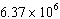km c.km b.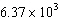km d.km

6.

Three values were obtained for the mass of a metal bar: 8.83 g; 8.84 g; 8.82 g. The known mass is 10.68 g. The values are
 a. accurate. c. both accurate and precise. b. precise. d. neither accurate nor precise.

7.

Calculate the following, and express the answer in scientific notation with the correct number of significant figures: 21.4 + 15 + 17.17 + 4.003
 a.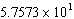b.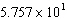c.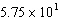d.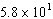8.

Calculate the following, and express the answer in scientific notation with the correct number of significant figures: 10.5 ´ 8.8 ´ 3.14
 a. 2900 b. 290.136 c.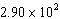d. 290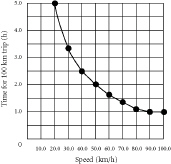9.

Which best describes this graphical relationship?
 a. direct b. indirect c. inverse d. impossible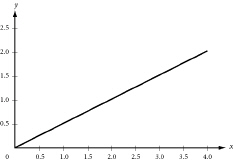10.

Which best describes this graphical relationship?
 a. impossible b. inverse c. indirect d. direct

11.

Which is the correct measurement for location marked by the arrow?a. 50.2 b. 51.8 c. 52 d. 51

12.

The accepted value is 29.35. Which correctly describes this student’s experimental data?
 Trial Measurement 1 29.48 2 28.97 3 29.27
 a. accurate but not precise c. both accurate and precise b. precise but not accurate d. neither accurate nor precise

13.

The accepted value is 1.43. Which correctly describes this student’s experimental data?
 Trial Measurement 1 1.29 2 1.93 3 0.88
 a. accurate but not precise c. both accurate and precise b. precise but not accurate d. neither accurate nor precise

14.

Which value has only 4 significant digits?
 a. 6.93 b. 0.045 c. 8450 d. 0.392

15.

Which is the sum of these values, to the appropriate number of significant digits?
17.358 + 3.502 + 20.14 =
 a. 41 b. 41 c. 41 d. 41

16.

Which is the product of these numbers, to the appropriate number of significant digits?
56.2
´ 9.2057 =
 a. 517 b. 517.4 c. 517.36 d. 517

17.

How many significant digits are in the value 530,405,000?
 a. 4 b. 5 c. 6 d. 9

18.

How many significant digits are in the value 0.0050340?
 a. 3 b. 5 c. 7 d. 8

19.

How many minutes are in 1 week?
 a. 168 min b. 1440 min c. 10 080 min d. 100 800 min

20.

If repeated measurements agree closely but differ widely from the accepted value, these measurements are
 a. neither precise nor accurate. b. accurate, but not precise. c. both precise and accurate. d. precise, but not accurate.

21.

The measurement 0.035550 g rounded off to two significant figures would be
 a. 0.03 g. b. 0.35 g. c. 0.036 g. d. 3.5 ´ 102 g.

22.

Which of these measurements has been expressed to three significant figures?
 a. 0.052 g b. 0.202 g c. 3.065 g d. 500 g

23.

Which of these measurements has been expressed to four significant figures?
 a. 0.0020 mm b. 0.0402 mm c. 30.00 mm d. 402.10 mm

24.

When 64.4 is divided by 2.00, the correct number of significant figures in the result is
 a. 1 b. 3 c. 4 d. 6

25.

How is the measurement 0.000 065 cm written in scientific notation?
 a. 65 ´ 10–6 cm c. 6.5 ´ 10–6 cm b. 6.5 ´ 10–5 cm d. 6.5 ´ 10–4 cm

26.

The speed of light is 300 000 km/s. In scientific notation, this speed is written to one significant figure as
 a. 3 ´ 105 km/s. c. 3. ´ 106 km/s. b. 3.0 ´ 105 km/s. d. 3.0 ´ 106 km/s.

27.

The closeness of a measurement to its true value is a measure of its ____.
 a. precision b. accuracy c. reproducibility d. usefulness

28.

In the measurement 0.503 L, which digit is the estimated digit?
 a. 5 c. 3 b. the 0 immediately to the left of the 3 d. the 0 to the left of the decimal point

29.

What is the temperature of absolute zero measured inC?
 a. –373C b. –273C c. –173C d. –73C

30.

If the temperature changes by 100 K, by how much does it change inC?
 a. 0C b. 37C c. 100C d. 273C